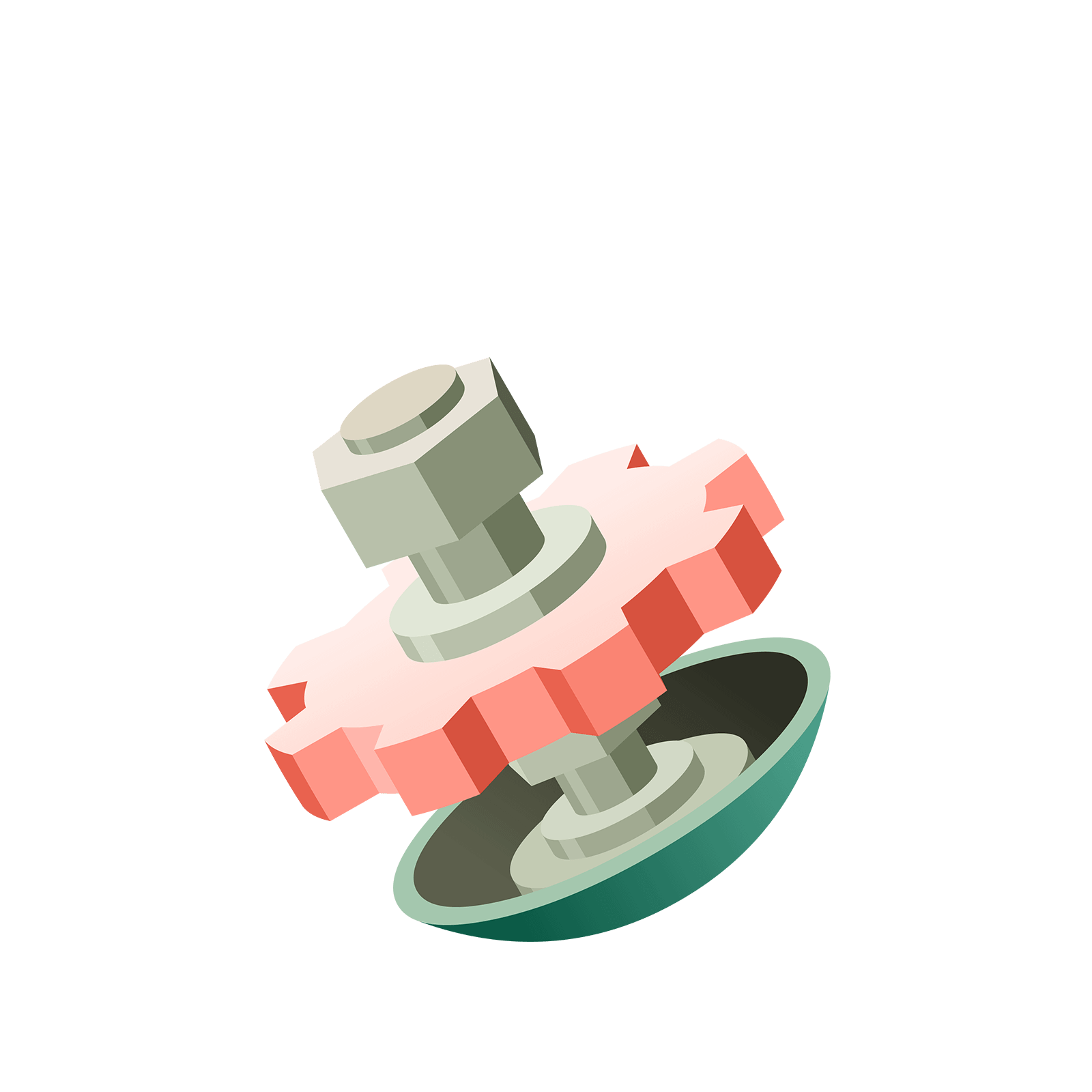06 103 624# Station  1The 1 ← 2 Machine

### Question 1

Put two dots in the rightmost box of the machine.### Question 2

Make those 2 dots explode!### Question 3

Stabilize the machine by making the dots explode.### Question 4

Find the $1 \leftarrow 2$ machine code for the number $4$.
Make the dots loaded in this machine explode until the machine becomes stable.
You will need to do more than one explosion!### Question 5

Make the dots explode as much as possible to find the code for the number $6$.### Question 6

Find the $1 \leftarrow 2$ machine code for the number $13$!### Question 7

Hint: You can erase dots as needed by moving them outside of the boxes.Add the dots to the rightmost box of the $1 \leftarrow 2$ machine to find which number has code $11001$.Wow! You have mastered the $1 \leftarrow 2$ machine!

Now you're ready for the $1 \leftarrow 3$ machine!